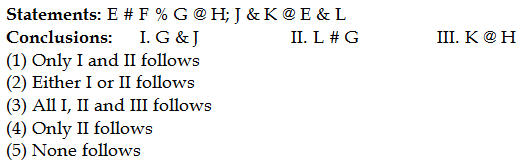## PREPARATORY QUESTIONS

### EQUAL-INEQUAL

#### PREPARATORY SET-15 (HARD)

Direction (Qs.1 to 5): In the following questions, the symbols &, @, #, \$ and % are used with the following meanings as illustrated below, answer the questions accordingly.

‘A & B’ means ‘A is not greater than B’
‘A @ B’ means ‘A is neither greater than nor equal to B’
‘A # B’ means ‘A is neither smaller than nor equal to B’
‘A \$ B’ means ‘A is not less than B’
‘A % B’ means ‘A is neither smaller than nor greater than B’

Now in each of the following questions assuming the given statement to be true, find which of the conclusions given below them is/are definitely true and give your answer accordingly.

Question No : 1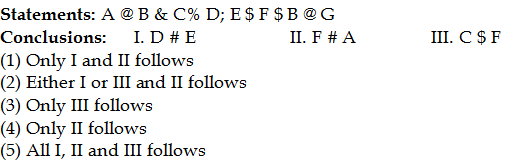Question No : 2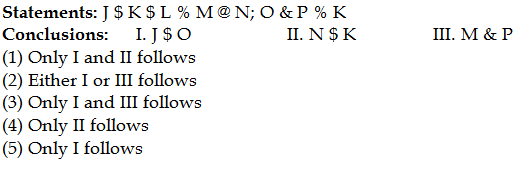Question No : 3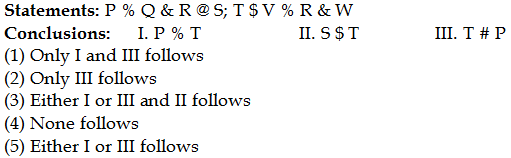Question No : 4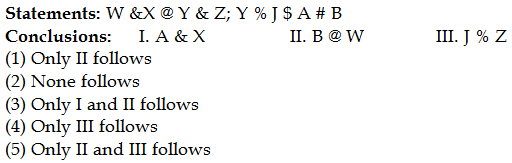Question No : 5